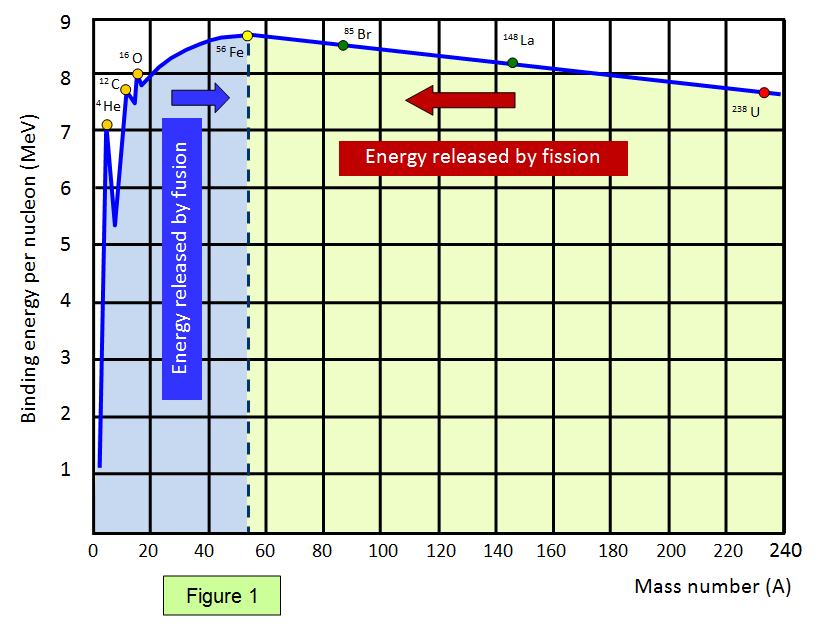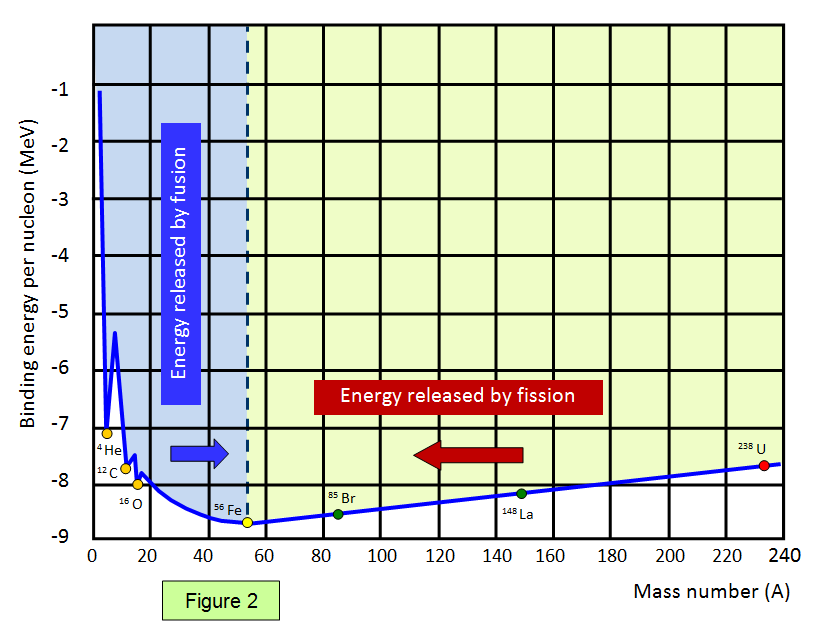# Binding energy per nucleon

Another useful quantity is the binding energy per nucleon. It can be defined simply as:

Binding Energy per nucleon = Binding Energy/ Nucleon Number

The graph below (Figure 1) shows the binding energy per nucleon against nucleon number. Elements with a high binding energy per nucleon are very difficult to break up. Iron 56 is close to the peak of the curve and has one of the highest binding energies per nucleon of any isotope.

(N.B the determination of the value of binding energy per nucleon for a given nucleus is a complex process and depends on the precise way it is calculated. Nickel 62, with a binding energy per nucleon of 8.7948 MeV exceeds that for iron 56 (8.7906 MeV).The part of the curve to the left shows that two light elements can produce energy by fusion while the part of the curve to the right shows that a heavy element can produce energy by fission. Notice that the diagram has been drawn with the binding energies per nucleon being shown as positive – this represents the energy needed to separate the particles.

Therefore if a reaction takes place where the products are closer to the base then the original nucleus (nuclei) then energy is given out.

For helium the binding energy per nucleon is 28.3/4 = 7.1 MeV.

The helium nucleus has a high binding energy per nucleon and is more stable than some of the other nuclei close to it in the periodic table.

Some of the binding energies per nucleon for some common elements are shown in the following table.

 Element Mass of nucleons (u) Nuclear mass (u) Binding energy (MeV) Binding energy per nucleon (MeV) Deuterium 2.01594 2.01355 2.23 1.12 Helium 4 4.03188 4.00151 28.29 7.07 Lithium 7 7.05649 7.01336 40.15 5.74 Beryllium 9 9.07243 9.00999 58.13 6.46 Iron 56 56.44913 55.92069 492.24 8.79 Silver 107 107.86187 106.87934 915.23 8.55 Iodine 127 128.02684 126.87544 1072.53 8.45 Lead 206 207.67109 205.92952 1622.27 7.88 Polonium 210 211.70297 209.93683 1645.16 7.83 Uranium 235 236.90849 234.99351 1783.80 7.59 Uranium 238 239.93448 238.00037 1801.63 7.57

A very useful web site containing a huge nuclear database is to be found at Nuclear data

It may be more helpful to consider the binding energy per nucleon diagram in the form shown in Figure 2 where reactions tend to move the nuclei towards the valley at the bottom of the curve. (In this case note that the binding energies per nucleon are given as negative values).© Keith Gibbs 2012